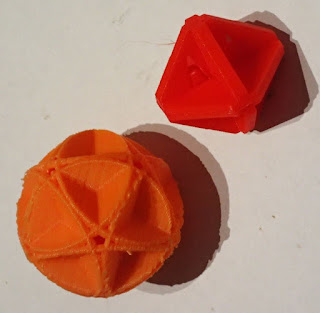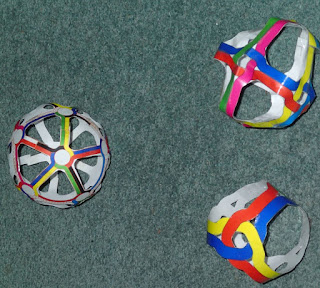# News and Events

Keep up to date with Steve Nurse's designs and 3d printing.

## Friday 8 July 2022

### Some models in context: skeleton octahedron etc.

Hi

A few weeks ago our friend Chris came over and introduced me to a type of cube, which I made a paper model of (fig 4 here ) After making a few different versions and variations of it, I realised some of the characteristics of the polyhedra that were being made:

* Models made from paper or flexible cane can represent the platonic solids. A basic version of these particular models have a ring around the outside of a face as the best representation of that face, like pictures at the bottom of the page here.

* These models have corners consisting of overlapping edge elements, and two canes or paper elements as edges.

* Because edges consist of 2 canes or strands joining each node, each node is "even", so by definition the network has 2 or no odd nodes, which is the condition for traversability. As well the network (no odd nodes) can make a Euler Circuit. Forming single circuits requires some twists in the edges, but more will follow on this later.

So there are node and edge variations of these polyhedra. This post concentrates on the node variations.

I've made a few edge net models from laminated paper (ie figs 5, 8, 14 here ) , and I thought I recognized an unusual polyhedron - the great dodecahedron - in the icosahedron model I'd made. (see this post also, I have drawn a great dodecaheron in 3d so knew what one was).

Then I saw another polyhedron that was new to me in what I'd already made, and I couldn't name it at the time. But on this page, fig 9 , and on this page, (figs 7 and 8 left) are versions of a skeletal octahedron, a polyhedron which in its pure, mathematical form has no volume. At that stage, I decided to map some of the work I've done, roughly as follows:

So the table goes through the platonic solids with rows corresponding to the number of edges meeting at nodes. Columns correspond to the turns that edge halves make as they go through the nodes. In the first column, edges join to form simple polygon faces. Intervening columns have overlap of faces. Lastly when the edges turn right around in the node, edges can merge. It should be possible to make all these forms in basket cane, and previous examples are figure 5 here (equivalent to figure 2 below), and figure 9 here, which is the skeletal octahedron.3d printed great dodecahedron and skeletal octahedronMade up models, top to bottom skeletal octahedron, great dodecahedron, tetrahedron.

Most of the rest of this post is the figures described in the table without too much wordage. Some of these have been posted before, but more or less as isolated things, and this is the first time I've placed them in context. This post is a "sketch", not an exhaustive "picture" so I hope you can work out what's going on.

Figure 1 shows an angle which corresponds to the angle in the table. Figure 3 shows 2 different node structures, the interlocking nodes can be put together 2 ways (see also figure 4) and the overlapping nodes 6 ways (or n!  / n factorial where n is the number of edges joining at the node). Figure 3 has a form that is slightly cheating, the tetrahedron is made up of sides defined by different-coloured edges, whereas the others show sides defined by same-coloured edges.

A version of this post with downloadable dwg and stl (3d printable) files is at https://www.thingiverse.com/thing:5427703 .

The octahedron and icosahedron sequences are nice!

Update Feb 2, 2023: I've put together an article on these structures, click this link to download.